Next: SLA_PLANET - Planetary Ephemerides
Up: SUBPROGRAM SPECIFICATIONS
Previous: SLA_PERTUE - Perturbed Universal Elements

## SLA_PLANEL - Planet Position from Elements

ACTION:
Heliocentric position and velocity of a planet, asteroid or comet, starting from orbital elements.

CALL:
CALL sla_PLANEL ( DATE, JFORM, EPOCH, ORBINC, ANODE, PERIH, AORQ, E, AORL, DM, PV, JSTAT)

GIVEN:

 D DATE Modified Julian Date (JD-2400000.5) JFORM I choice of element set (1-3, see Note 3, below) EPOCH D epoch of elements (t0 or T, TT MJD) ORBINC D inclination (i, radians) ANODE D longitude of the ascending node (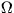, radians) PERIH D longitude or argument of perihelion (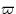or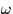, radians) AORQ D mean distance or perihelion distance (a or q, AU) E D eccentricity (e) AORL D mean anomaly or longitude (M or L, radians, JFORM=1,2 only) DM D daily motion (n, radians, JFORM=1 only)

RETURNED:

 D(6) PV heliocentric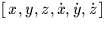, equatorial, J2000 (AU, AU/s) JSTAT I status: 0 = OK -1 = illegal JFORM -2 = illegal E -3 = illegal AORQ -4 = illegal DM -5 = numerical error

NOTES:
1.
DATE is the instant for which the prediction is required. It is in the TT timescale (formerly Ephemeris Time, ET) and is a Modified Julian Date (JD-2400000.5).
2.
The elements are with respect to the J2000 ecliptic and equinox.
3.
Three different element-format options are available, as follows.

JFORM=1, suitable for the major planets:


EPOCH 		 = 		 epoch of elements t0 (TT MJD)
ANODE 		 = 		 longitude of the ascending node(radians)
PERIH 		 = 		 longitude of perihelion(radians)
AORQ 		 = 		 mean distance a (AU)
E 		 = 		 eccentricity e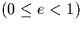AORL 		 = 		 mean longitude L (radians)
DM 		 = 		 daily motion n (radians)


JFORM=2, suitable for minor planets:


EPOCH 		 = 		 epoch of elements t0 (TT MJD)
ANODE 		 = 		 longitude of the ascending node(radians)
PERIH 		 = 		 argument of perihelion(radians)
AORQ 		 = 		 mean distance a (AU)
E 		 = 		 eccentricity eAORL 		 = 		 mean anomaly M (radians)


JFORM=3, suitable for comets:


EPOCH 		 = 		 epoch of perihelion T (TT MJD)
ANODE 		 = 		 longitude of the ascending node(radians)
PERIH 		 = 		 argument of perihelion(radians)
AORQ 		 = 		 perihelion distance q (AU)
E 		 = 		 eccentricity e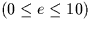4.
Unused elements (DM for JFORM=2, AORL and DM for JFORM=3) are not accessed.
5.
The reference frame for the result is equatorial and is with respect to the mean equinox and ecliptic of epoch J2000.
6.
The algorithm was originally adapted from the EPHSLA program of D.H.P.Jones (private communication, 1996). The method is based on Stumpff's Universal Variables.

REFERENCE:
Everhart, E. & Pitkin, E.T., Am. J. Phys. 51, 712, 1983.Next: SLA_PLANET - Planetary Ephemerides
Up: SUBPROGRAM SPECIFICATIONS
Previous: SLA_PERTUE - Perturbed Universal Elements

SLALIB --- Positional Astronomy Library